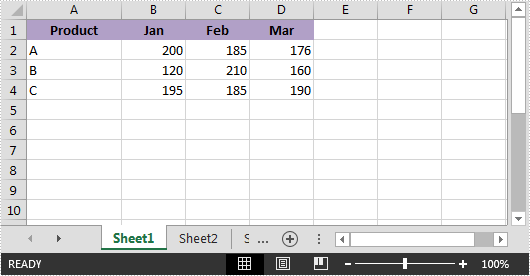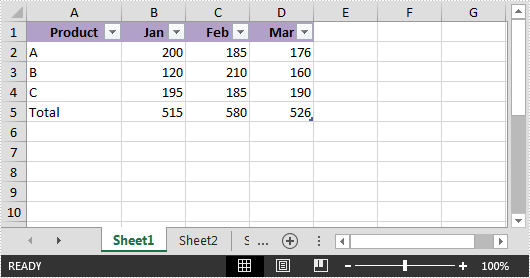# Add a Total Row to Table in Excel in C#

We can quickly total data in an Excel table by adding a total row. This article demonstrates how to add a total row to a table in Excel using Spire.XLS.

Blow is the screenshot of the example file:Detail steps:

Step 1: Instantiate a Workbook object and load the excel file.

```Workbook workbook = new Workbook();
```

Step 2: Get the first worksheet.

```Worksheet sheet = workbook.Worksheets;
```

Step 3: Create a table with the data from the specific cell range.

```IListObject table = sheet.ListObjects.Create("Table", sheet.Range["A1:D4"]);
```

Step 4: Display total row.

```table.DisplayTotalRow = true;
```

Step 5: Add a total row.

```table.Columns.TotalsRowLabel = "Total";
table.Columns.TotalsCalculation = ExcelTotalsCalculation.Sum;
table.Columns.TotalsCalculation = ExcelTotalsCalculation.Sum;
table.Columns.TotalsCalculation = ExcelTotalsCalculation.Sum;
```

Step 6: Save the file.

```workbook.SaveToFile("AddTotalRow.xlsx", ExcelVersion.Version2013);
```

Output:Full code:

```using Spire.Xls;
using Spire.Xls.Core;
{
class Program
{
static void Main(string[] args)
{
//Instantiate a Workbook object
Workbook workbook = new Workbook();

//Get the first worksheet
Worksheet sheet = workbook.Worksheets;

//Create a table with the data from the specific cell range
IListObject table = sheet.ListObjects.Create("Table", sheet.Range["A1:D4"]);

//Display total row
table.DisplayTotalRow = true;